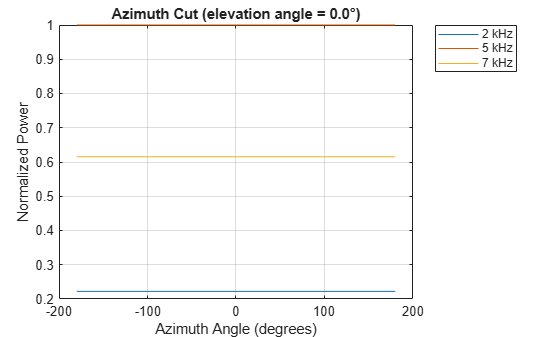Documentation

# step

System object: phased.IsotropicProjector
Package: phased

Voltage response of isotropic projector

## Syntax

```resp = step(projector,freq,ang) ```

## Description

### Note

Instead of using the `step` method to perform the operation defined by the System object™, you can call the object with arguments, as if it were a function. For example, ```y = step(obj,x)``` and `y = obj(x)` perform equivalent operations.

`resp = step(projector,freq,ang)` returns the voltage response for the projector at the specified operating frequencies and in the specified directions of arriving signals.

### Note

The object performs an initialization the first time the object is executed. This initialization locks nontunable properties (MATLAB) and input specifications, such as dimensions, complexity, and data type of the input data. If you change a nontunable property or an input specification, the System object issues an error. To change nontunable properties or inputs, you must first call the `release` method to unlock the object.

## Input Arguments

expand all

Isotropic projector, specified as a `phased.IsotropicProjector` System object.

Example: `phased.IsotropicProjector`

Voltage response frequencies of projector, specified as a positive real scalar or a real-valued 1-by-L vector of positive values. Units are in Hz.

Data Types: `double`

Direction of arriving signals, specified as a real-valued 1-by-M row vector or 2-by-M matrix. When `ang` is a 2-by-M matrix, each column of the matrix specifies the direction in the form `[azimuth;elevation]`. The azimuth angle must lie between –180° and 180°, inclusive. The elevation angle must lie between –90° and 90°, inclusive.

When `ang` is a 1-by-M row vector, each element specifies the azimuth angle of the arriving signal. In this case, the corresponding elevation angle is assumed to be zero.

Data Types: `double`

## Output Arguments

expand all

Voltage response of projector, returned as a real-valued M-by-L matrix. M represents the number of angles specified in `ang`, and L represents the number of frequencies specified in `freq`. Units are in V/Pa.

## Examples

expand all

Examine the response and patterns of an isotropic projector operating between 1 kHz and 10 kHz.

Set the projector parameters and obtain the voltage response at five different elevation angles: -30°, -15°, 0°, 15° and 30&deg. All elevation angles at 0° azimuth angle. The voltage response is computed at 2 kHz.

```projector = phased.IsotropicProjector('FrequencyRange',[1,10]*1e3); fc = 2e3; resp = projector(fc,[0,0,0,0,0;-30,-15,0,15,30]);```

Draw a 3-D plot of the voltage response.

```pattern(projector,fc,[-180:180],[-90:90],'CoordinateSystem','polar', ... 'Type','power')```Examine the response and patterns of an isotropic projector at three different frequencies. The projector operates between 1 kHz and 10 kHz. Specify the voltage response as a vector.

Set up the projector parameters, and obtain the voltage response at 45° azimuth and 30° elevation. Compute the responses at signal frequencies of 2, 5, and 7 kHz.

```projector = phased.IsotropicProjector('FrequencyRange',[1 10]*1e3, ... 'VoltageResponse',[90 95 100 95 90]); fc = [2e3 5e3 7e3]; resp = projector(fc,[45;30]); resp```
```resp = 1×3 0.0426 0.0903 0.0708 ```

Next, draw a 2-D plot of the voltage response as a function of azimuth

```pattern(projector,fc,[-180:180],0,'CoordinateSystem','rectangular', ... 'Type','power')```## Algorithms

The total response of a projector is a combination of its frequency response and spatial response. `phased.IsotropicProjector` calculates both responses using nearest neighbor interpolation, and then multiplies the responses to form the total response.

## See Also

#### Hybrid Beamforming for Massive MIMO Phased Array Systems

Download the white paper